# Process Text using TFIDF in PythonComputers are good with numbers, but not that much with textual data. One of the most widely used techniques to process textual data is TF-IDF. In this article, we will learn how it works and what are its features.

From our intuition, we think that the words which appear more often should have a greater weight in textual data analysis, but that’s not always the case. Words such as “the”, “will”, and “you” — called stopwords — appear the most in a corpus of text, but are of very little significance. Instead, the words which are rare are the ones that actually help in distinguishing between the data, and carry more weight.

### An introduction to TF-IDF

TF-IDF stands for “Term Frequency — Inverse Data Frequency”. First, we will learn what this term means mathematically.

Term Frequency (tf): gives us the frequency of the word in each document in the corpus. It is the ratio of number of times the word appears in a document compared to the total number of words in that document. It increases as the number of occurrences of that word within the document increases. Each document has its own tf.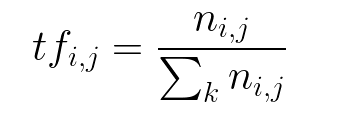Inverse Data Frequency (idf): used to calculate the weight of rare words across all documents in the corpus. The words that occur rarely in the corpus have a high IDF score. It is given by the equation below.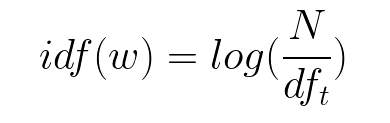Combining these two we come up with the TF-IDF score (w) for a word in a document in the corpus. It is the product of tf and idf: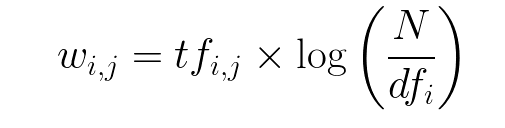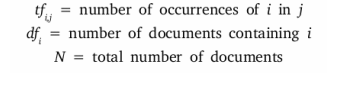Let’s take an example to get a clearer understanding.

Sentence 1 : The car is driven on the road.

Sentence 2: The truck is driven on the highway.

In this example, each sentence is a separate document.

We will now calculate the TF-IDF for the above two documents, which represent our corpus.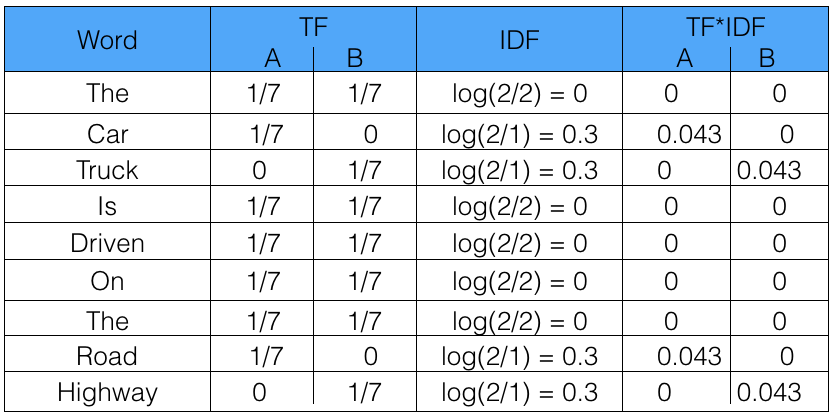From the above table, we can see that TF-IDF of common words was zero, which shows they are not significant. On the other hand, the TF-IDF of “car” , “truck”, “road”, and “highway” are non-zero. These words have more significance.

### Using Python to calculate TF-IDF

Lets now code TF-IDF in Python from scratch. After that, we will see how we can use sklearn to automate the process.

The function `computeTF` computes the TF score for each word in the corpus, by document.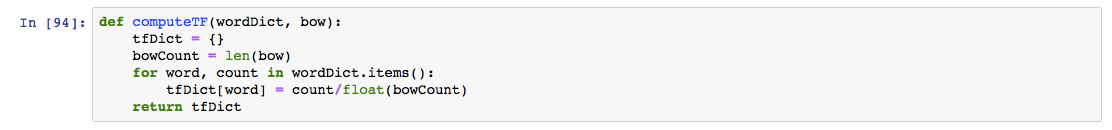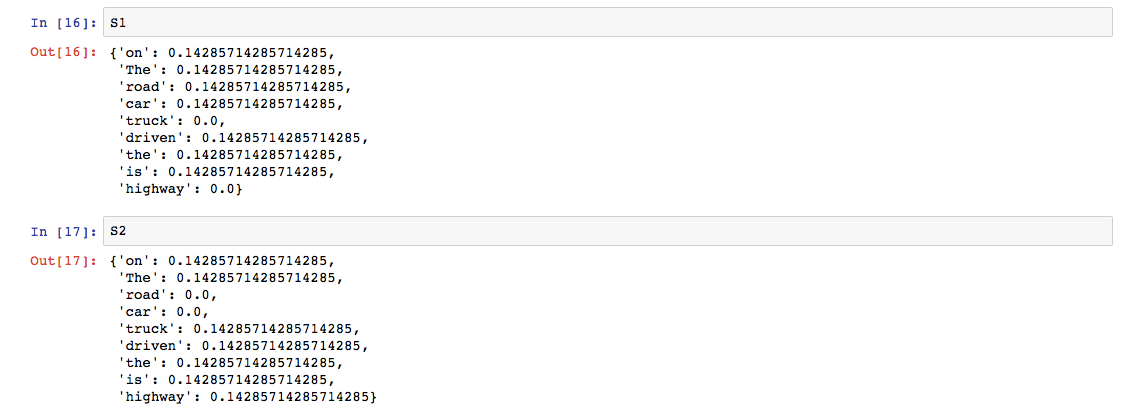The function `computeIDF` computes the IDF score of every word in the corpus.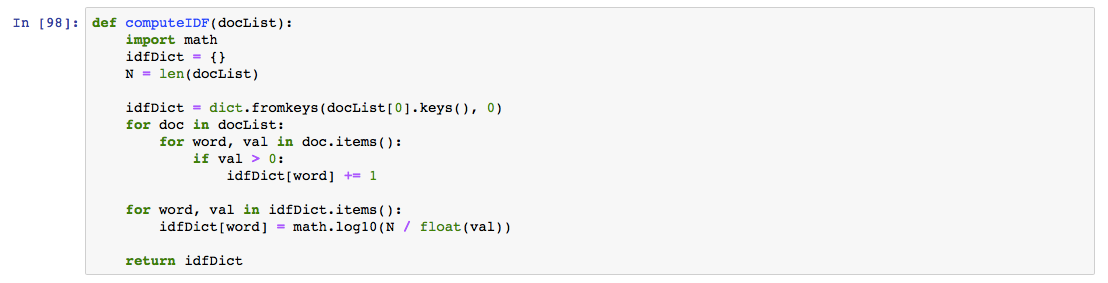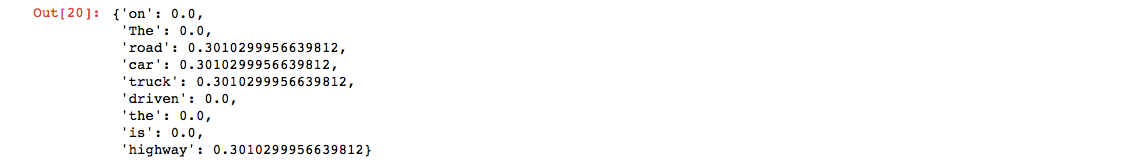The function `computeTFIDF` below computes the TF-IDF score for each word, by multiplying the TF and IDF scores.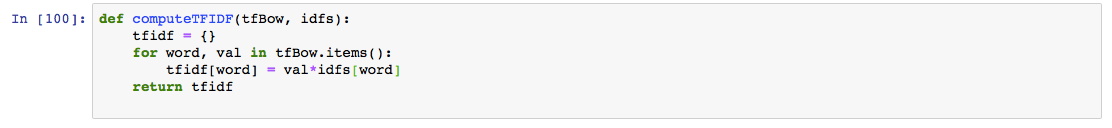The output produced by the above code for the set of documents D1 and D2 is the same as what we manually calculated above in the table.You can refer to this link for the complete implementation.

#### sklearn

Now we will see how we can implement this using sklearn in Python.

First, we will import `TfidfVectorizer` from `sklearn.feature_extraction.text`: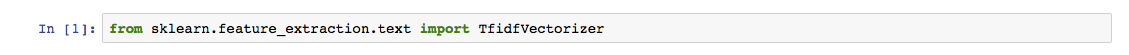Now we will initialise the `vectorizer` and then call fit and transform over it to calculate the TF-IDF score for the text.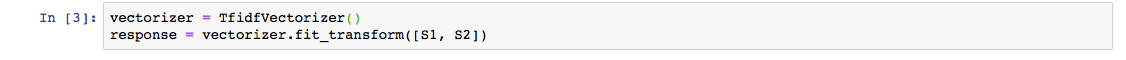Under the hood, the sklearn fit_transform executes the following `fit` and `transform` functions. These can be found in the official sklearn library at GitHub.

``````    def fit(self, X, y=None):
"""Learn the idf vector (global term weights)
Parameters
----------
X : sparse matrix, [n_samples, n_features]
a matrix of term/token counts
"""
if not sp.issparse(X):
X = sp.csc_matrix(X)
if self.use_idf:
n_samples, n_features = X.shape
df = _document_frequency(X)

# perform idf smoothing if required
df += int(self.smooth_idf)
n_samples += int(self.smooth_idf)

# log+1 instead of log makes sure terms with zero idf don't get
# suppressed entirely.
idf = np.log(float(n_samples) / df) + 1.0
self._idf_diag = sp.spdiags(idf, diags=0, m=n_features,
n=n_features, format='csr')

return self

def transform(self, X, copy=True):
"""Transform a count matrix to a tf or tf-idf representation
Parameters
----------
X : sparse matrix, [n_samples, n_features]
a matrix of term/token counts
copy : boolean, default True
Whether to copy X and operate on the copy or perform in-place
operations.
Returns
-------
vectors : sparse matrix, [n_samples, n_features]
"""
if hasattr(X, 'dtype') and np.issubdtype(X.dtype, np.floating):
# preserve float family dtype
X = sp.csr_matrix(X, copy=copy)
else:
# convert counts or binary occurrences to floats
X = sp.csr_matrix(X, dtype=np.float64, copy=copy)

n_samples, n_features = X.shape

if self.sublinear_tf:
np.log(X.data, X.data)
X.data += 1

if self.use_idf:
check_is_fitted(self, '_idf_diag', 'idf vector is not fitted')

expected_n_features = self._idf_diag.shape
if n_features != expected_n_features:
raise ValueError("Input has n_features=%d while the model"
" has been trained with n_features=%d" % (
n_features, expected_n_features))
# *= doesn't work
X = X * self._idf_diag

if self.norm:
X = normalize(X, norm=self.norm, copy=False)

return X
``````

One thing to notice in the above code is that, instead of just the log of n_samples, 1 has been added to n_samples to calculate the IDF score. This ensures that the words with an IDF score of zero don’t get suppressed entirely.

The output obtained is in the form of a skewed matrix, which is normalised to get the following result.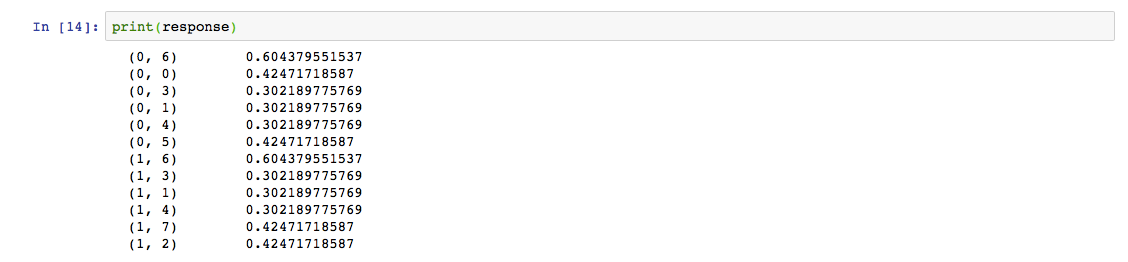Thus we saw how we can easily code TF-IDF in just 4 lines using sklearn. Now we understand how powerful TF-IDF is as a tool to process textual data out of a corpus. To learn more about sklearn TF-IDF, you can use this link.

Happy coding!